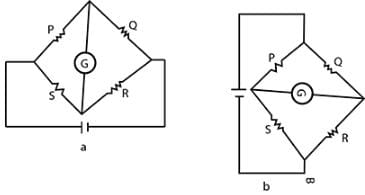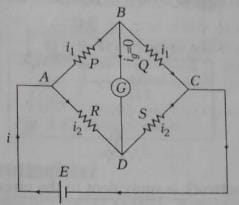NEET  >  Test: Electrical Appliances

# Test: Electrical Appliances

Test Description

## 10 Questions MCQ Test Topic-wise MCQ Tests for NEET | Test: Electrical Appliances

Test: Electrical Appliances for NEET 2022 is part of Topic-wise MCQ Tests for NEET preparation. The Test: Electrical Appliances questions and answers have been prepared according to the NEET exam syllabus.The Test: Electrical Appliances MCQs are made for NEET 2022 Exam. Find important definitions, questions, notes, meanings, examples, exercises, MCQs and online tests for Test: Electrical Appliances below.
Solutions of Test: Electrical Appliances questions in English are available as part of our Topic-wise MCQ Tests for NEET for NEET & Test: Electrical Appliances solutions in Hindi for Topic-wise MCQ Tests for NEET course. Download more important topics, notes, lectures and mock test series for NEET Exam by signing up for free. Attempt Test: Electrical Appliances | 10 questions in 10 minutes | Mock test for NEET preparation | Free important questions MCQ to study Topic-wise MCQ Tests for NEET for NEET Exam | Download free PDF with solutions
 1 Crore+ students have signed up on EduRev. Have you?
Test: Electrical Appliances - Question 1

### Sensitivity of potentiometer can be increased by

Detailed Solution for Test: Electrical Appliances - Question 1

Sensitivity of the potentiometer means the smallest potential difference it can measure. It can be increased by reducing the potential gradient. The same is possible by increasing the length of the potentiometer. Hence the correct option is option C.

Test: Electrical Appliances - Question 2

### In a potentiometer a cell of e.m.f 2V gives a balance point at 30cm. If the cell is replaced by another cell and the balance point shifts to 60cm.What is the e.m.f of the other cell?​

Detailed Solution for Test: Electrical Appliances - Question 2

Changes(if required):
Solution:
We can use the formula E1/E2=L1/L2
E1=2v,E2=?,l1=30,l2=60
2/E2=30/60
E2=120/30=4v

Test: Electrical Appliances - Question 3

### When the position of cell and galvanometer in a Wheatstone bridge is inter-changed, its balanced condition

Detailed Solution for Test: Electrical Appliances - Question 3

For balanced Wheatstone bridge which is shown in figure a, P/Q​=S/R​
If we interchange the cell and galvanometer then circuit becomes as shown in figure b.
and balanced condition, P/S​=Q​/R⇒P/Q​=S/R​
Thus, balanced point remains unchanged.Test: Electrical Appliances - Question 4

An electric bulb is rated 220 V and 100 W. Power consumed by it operated on 110 V is

Detailed Solution for Test: Electrical Appliances - Question 4

Power, P= V2/R​
R= V2/P​
R=220×220/100 ​=484Ω
The current flow in the circuit,
i= V′/R​ (V′= supplied voltage)
i=110/484​=0.2272A
The voltage drop across the bulb is 110V
The power consumed by the bulb,
Pb​=V2/R​=110×110​/484
Pb​=25W
The correct option is C.

Test: Electrical Appliances - Question 5

Meter Bridge or Slide Wire Bridge is a practical form of

Detailed Solution for Test: Electrical Appliances - Question 5

The meter bridge principle is based on the Wheatstone bridge circuit which says that if at any point of(of a wire), the ratio of two resistances (say R1) is equal to the ratio of another two resistance (say R3 and R4 where R4is the unknown resistance),Then there shall be no flow of current at that point between those points and the edge containing the resistances (R1/R2 and R3/R4) therefore, applying it to the meter bridge, at such point, the galvanometer will show zero defection.

Test: Electrical Appliances - Question 6

When Wheatstone bridge is in balance condition, the current through galvanometer will be

Detailed Solution for Test: Electrical Appliances - Question 6The bridge is said to be balanced when deflection in galvanometer is zero(Ig=0), i.e., no current flows through the galvanometer(branch BD).In the balanced condition,
P/Q=R/S
On mutually changing the position of the cell and galvanometer, this condition will not change.

Test: Electrical Appliances - Question 7

The resistance in ohms in the four arms of a Wheatstone bridge are as follows. In which combination of the following is the bridge balanced?​

Detailed Solution for Test: Electrical Appliances - Question 7

Wheat stone bridge follows

P/Q = R/S

So 2/3 = 4/6

Test: Electrical Appliances - Question 8

Two bulbs A and B are rated 100 W, 120 V and 10 W, 120 V respectively. They are connected across a 120 V source in series. Calculate the current through each bulb.​

Detailed Solution for Test: Electrical Appliances - Question 8

Bulb A,
PA=100w
VA=120v
We know that, P=VI
So, I=P/V=100w/120v=5/6A=0.83A
Bulb B,
PB=100w
VB=120v
We know that, P=VI
So, I=P/V=10w/120v=1/12A=0.083A
Bulb A will consume more energy than bulb B when they are connected in parallel.

Test: Electrical Appliances - Question 9

Wheatstone bridge is used to measure

Detailed Solution for Test: Electrical Appliances - Question 9

When the variable resistor is adjusted, then the current in the galvanometer becomes zero, the ratio of two two unknown resistors is equal to the ratio of value of unknown resistance and adjusted value of variable resistance. By using a Wheatstone Bridge the unknown electrical resistance value can easily measure.

Test: Electrical Appliances - Question 10

The Wheatstone bridge and its balance condition provides a practical method for the determination of

Detailed Solution for Test: Electrical Appliances - Question 10

A wheatstone bridge is an electrical bridge consisting of two branches of a parallel circuit joined by a galvanometer and used for determining the value of an unknown resistance in one of the branches.

## Topic-wise MCQ Tests for NEET

1493 tests
 Use Code STAYHOME200 and get INR 200 additional OFF Use Coupon Code
Information about Test: Electrical Appliances Page
In this test you can find the Exam questions for Test: Electrical Appliances solved & explained in the simplest way possible. Besides giving Questions and answers for Test: Electrical Appliances, EduRev gives you an ample number of Online tests for practice

## Topic-wise MCQ Tests for NEET

1493 tests

### How to Prepare for NEET

Read our guide to prepare for NEET which is created by Toppers & the best Teachers